Download Presentation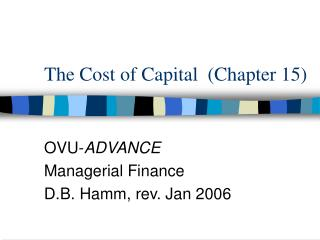The Cost of Capital (Chapter 15)

# The Cost of Capital (Chapter 15) - PowerPoint PPT Presentation

The Cost of Capital (Chapter 15). OVU- ADVANCE Managerial Finance D.B. Hamm, rev. Jan 2006. “Cost of Capital?”. When we say a firm has a “cost of capital” of, for example, 12%, we are saying: The firm can only have a positive NPV on a project if return exceeds 12%I am the owner, or an agent authorized to act on behalf of the owner, of the copyrighted work described.
Download Presentation## The Cost of Capital (Chapter 15)

An Image/Link below is provided (as is) to download presentation

Download Policy: Content on the Website is provided to you AS IS for your information and personal use and may not be sold / licensed / shared on other websites without getting consent from its author.While downloading, if for some reason you are not able to download a presentation, the publisher may have deleted the file from their server.

- - - - - - - - - - - - - - - - - - - - - - - - - - E N D - - - - - - - - - - - - - - - - - - - - - - - - - -
Presentation Transcript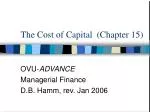### The Cost of Capital (Chapter 15)

Managerial Finance

D.B. Hamm, rev. Jan 2006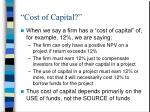“Cost of Capital?”
• When we say a firm has a “cost of capital” of, for example, 12%, we are saying:
• The firm can only have a positive NPV on a project if return exceeds 12%
• The firm must earn 12% just to compensate investors for the use of their capital in a project
• The use of capital in a project must earn 12% or more, not that it will necessarily cost 12% to borrow funds for the project
• Thus cost of capital depends primarily on the USE of funds, not the SOURCE of funds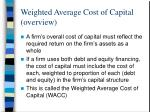Weighted Average Cost of Capital (overview)
• A firm’s overall cost of capital must reflect the required return on the firm’s assets as a whole
• If a firm uses both debt and equity financing, the cost of capital must include the cost of each, weighted to proportion of each (debt and equity) in the firm’s capital structure
• This is called the Weighted Average Cost of Capital (WACC)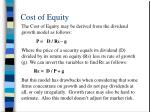Cost of Equity

The Cost of Equity may be derived from the dividend growth model as follows:

P = D / RE – g

Where the price of a security equals its dividend (D) divided by its return on equity (RE) less its rate of growth (g). We can invert the variables to find RE as follows:

RE = D / P + g

But this model has drawbacks when considering that some firms concentrate on growth and do not pay dividends at all, or only irregularly. Growth rates may also be hard to estimate. Also this model doesn’t adjust for market risk.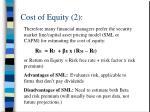Cost of Equity (2):

Therefore many financial managers prefer the security market line/capital asset pricing model (SML or CAPM) for estimating the cost of equity:

RE = Rf + βE x (RM – Rf)

or Return on Equity = Risk free rate + (risk factor x risk premium)

Advantages of SML: Evaluates risk, applicable to firms that don’t pay dividends

Disadvantages of SML: Need to estimate both Beta and risk premium (will usually base on past data, not future projections.)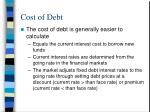Cost of Debt
• The cost of debt is generally easier to calculate
• Equals the current interest cost to borrow new funds
• Current interest rates are determined from the going rate in the financial markets
• The market adjusts fixed debt interest rates to the going rate through setting debt prices at a discount (current rate > than face rate) or premium (current rate < than face rate)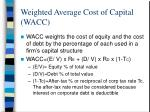Weighted Average Cost of Capital (WACC)
• WACC weights the cost of equity and the cost of debt by the percentage of each used in a firm’s capital structure
• WACC=(E/ V) x RE + (D/ V) x RD x (1-TC)
• (E/V)= Equity % of total value
• (D/V)=Debt % of total value
• (1-Tc)=After-tax % or reciprocal of corp tax rate Tc. The after-tax rate must be considered because interest on corporate debt is deductible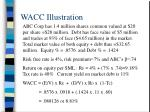WACC Illustration

ABC Corp has 1.4 million shares common valued at \$20 per share =\$28 million. Debt has face value of \$5 million and trades at 93% of face (\$4.65 million) in the market. Total market value of both equity + debt thus =\$32.65 million. Equity % = .8576 and Debt % = .1424

Risk free rate is 4%, risk premium=7% and ABC’s β=.74

Return on equity per SML : RE = 4% + (7% x .74)=9.18% Tax rate is 40% Current yield on market debt is 11%

WACC = (E/V) x RE + (D/V) x RD x (1-Tc)

= .8576 x .0918 + (.1424 x .11 x .60)

= .088126 or 8.81%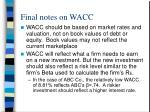Final notes on WACC
• WACC should be based on market rates and valuation, not on book values of debt or equity. Book values may not reflect the current marketplace
• WACC will reflect what a firm needs to earn on a new investment. But the new investment should also reflect a risk level similar to the firm’s Beta used to calculate the firm’s RE.
• In the case of ABC Co., the relatively low WACC of 8.81% reflects ABC’s β=.74. A riskier investment should reflect a higher interest rate.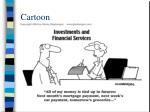### Financial Leverage (Chapter 17)

Managerial Finance

D.B. Hamm, Jan. 2006Equity vs Debt Financing (1)
• Since the WACC is the weighted average of cost of equity + cost of debt, we can vary the WACC by changing the mix of debt + equity
• If cost of debt < cost of equity, we can reduce WACC by increasing the % of debt in the mix and vice versa
• The value of the firm (its earning’s potential) is maximized when its WACC is minimized.
• A firm with a lower cost of capital can more easily return profits to its ownersDebt vs Equity Financing (2):
• The optimal, or target capital structure is the structure with the lowest possible WACC
• The Interest Tax Shield (deductibility of corp. interest) is critical here, because it effectively lowers the cost of debt.
• Therefore for many firms, the use of financial leverage (debt financing) can lower WACC and increase profitabilityDebt vs. Equity Financing (3):
• Warning: choice between debt & equity can not be based on interest rates, etc. alone. Risk must be considered as well
• Systematic risk (see ch. 13) consists of two factors which must be considered
• Business risk—risk inherent in firm’s operations
• Financial risk—risk inherent in using debt financing
• Remember debt is a multiplier:
• it can multiply returns if returns > cost of debt; but
• it can also multiply losses, or returns < cost of debt.Financial Leverage Considerations:
• If profits are down, dividends (the key cost of equity financing) can often be deferred.
• Interest (cost of debt) must always be paid for a firm to remain solvent
• Financial distress costs: costs incurred with going bankrupt or costs that must be paid to avoid bankruptcy
• According to the static theory of capital structure, gains from the tax shield are offset by the greater potential of financial distress costs.Optimal Capital Structure:
• Optimal capital structure is achieved by finding the point at which the tax benefit of an extra dollar of debt = potential cost of financial distress. This is the point of:
• Optimal amount of debt
• Maximum value of the firm
• Optimal debt to equity ratio
• Minimal cost of WACC
• This will obviously vary from firm to firm and takes some effort to evaluate. No single equation can guarantee profitability or even survivalCritical considerations:
• Firms with greater risk of financial distress must borrow less
• The greater volatility in EBIT, the less a firm should borrow (magnify risk of losses)
• Costs of financial distress can be minimized the more easily firm assets can be liquidated to cover obligations
• A firm with more liquid assets may therefore have less financial risk in borrowing
• A firm with more proprietary assets (unique to the firm, hard to liquidate) should minimize borrowingCongratulations!

You are now all financial wizards!

End of module!